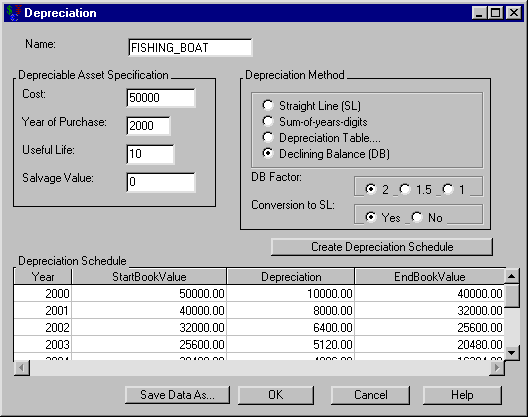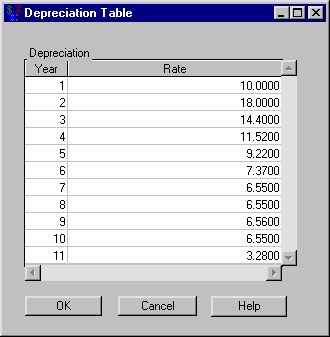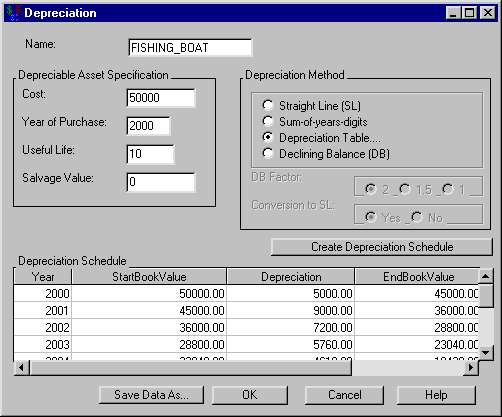# Investments

### Depreciation Tasks

Subsections:

Commercial assets are considered to lose value as time passes. For tax purposes, you want to quantify this loss. This investment structure helps calculate appropriate values.

Suppose you spend \$50,000 for a commercial fishing boat that is considered to have a ten-year useful life. How would you depreciate it?

In the Investment Analysis dialog box, select InvestmentNewDepreciation from the menu bar to open the Depreciation dialog box.

#### Specifying Depreciation Terms to Create a Depreciation Table

To specify the depreciation, follow these steps:

1. Enter FISHING_BOAT for the Name.

2. Enter 50000 for the Cost.

3. Enter 2000 for the Year of Purchase.

4. Enter 10 for the Useful Life.

5. Enter 0 for the Salvage Value.

You must specify the depreciation before generating the depreciation schedule. After you have specified the depreciation, click Create Depreciation Schedule to generate a depreciation schedule like the one displayed in Figure 56.10.

Figure 56.10: Creating a Depreciation ScheduleThe default depreciation method is Declining Balance (with Conversion to Straight Line). Try the following methods to see how they each affect the schedule:

• Straight Line

• Sum-of-years Digits

• Declining Balance (without conversion to Straight Line)

It might be useful to compare the value of the boat at 5 years for each method.

A description of these methods is available in the section Depreciation Methods.

#### Using the Depreciation Table

Sometimes you want to force the depreciation rates to be certain percentages each year. This option is particularly useful for calculating modified accelerated cost recovery system (MACRS) depreciations. The United States’ Tax Reform Act of 1986 set depreciation rates for an asset based on an assumed lifetime for that asset. Since these lists of rates are important to many people, Investment Analysis provides SAS data sets for situations with yearly rates (using the "half-year convention"). Find them at SASHELP.MACRS* where * refers to the class of the property. For example, use SASHELP.MACRS15 for a fifteen-year property. (When using the MACRS with the Tax Reform Act tables, you must set the Salvage Value to zero.)

Suppose you want to compute the depreciation schedule for the commercial fishing boat described in the section Depreciation Tasks. The boat is a ten-year property according to the Tax Reform Act of 1986.

To employ the MACRS depreciation from the Depreciation dialog box, follow these steps:

1. Select the Depreciation Table option within the Depreciation Method area and click OK. This opens the Depreciation Table dialog box.

2. Right-click within the Depreciation area (which pops up a menu) and select Load.

3. Enter SASHELP.MACRS10 for the Dataset Name. The dialog box should look like Figure 56.11.

Figure 56.11: MACRS Percentages for a Ten-Year PropertyClick OK to return to the Depreciation dialog box. Click Create Depreciation Schedule and the depreciation schedule fills (see Figure 56.12).

Note there are eleven entries in this depreciation schedule. This is because of the half-year convention that enables you to deduct one half of a year the first year which leaves a half year to deduct after the useful life is over.

Figure 56.12: Depreciation Table with MACRS10Click OK to return to the Investment Analysis dialog box.